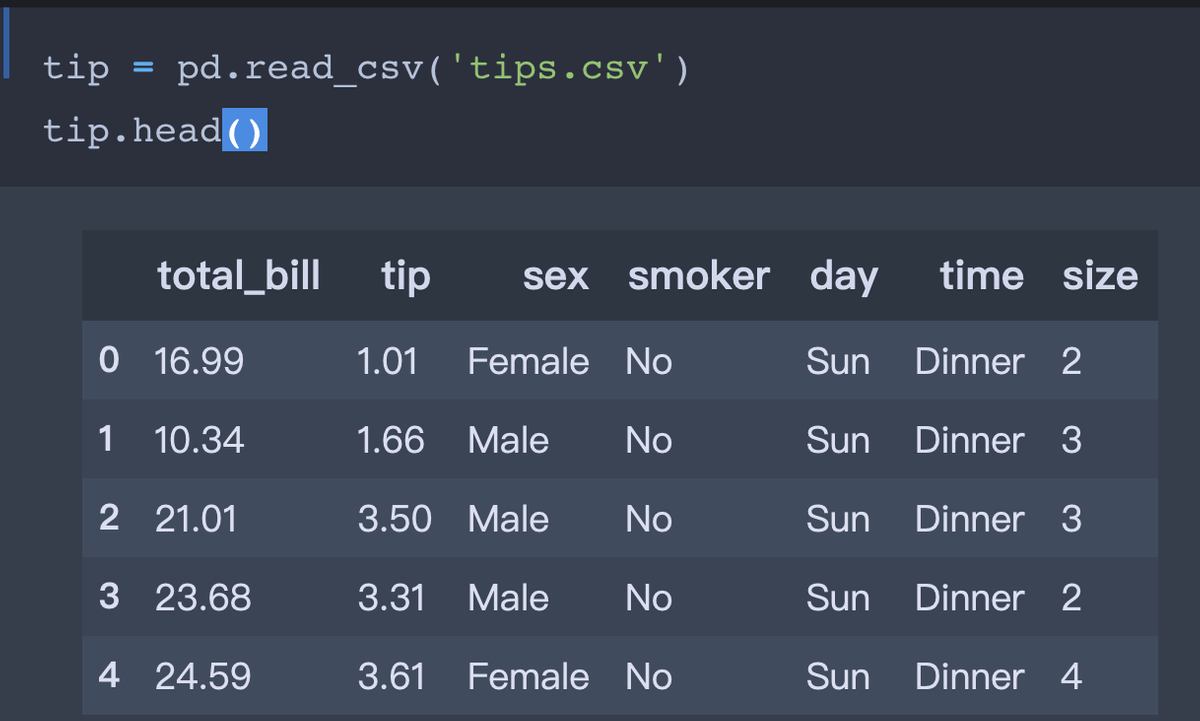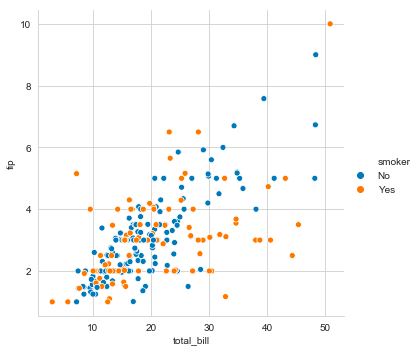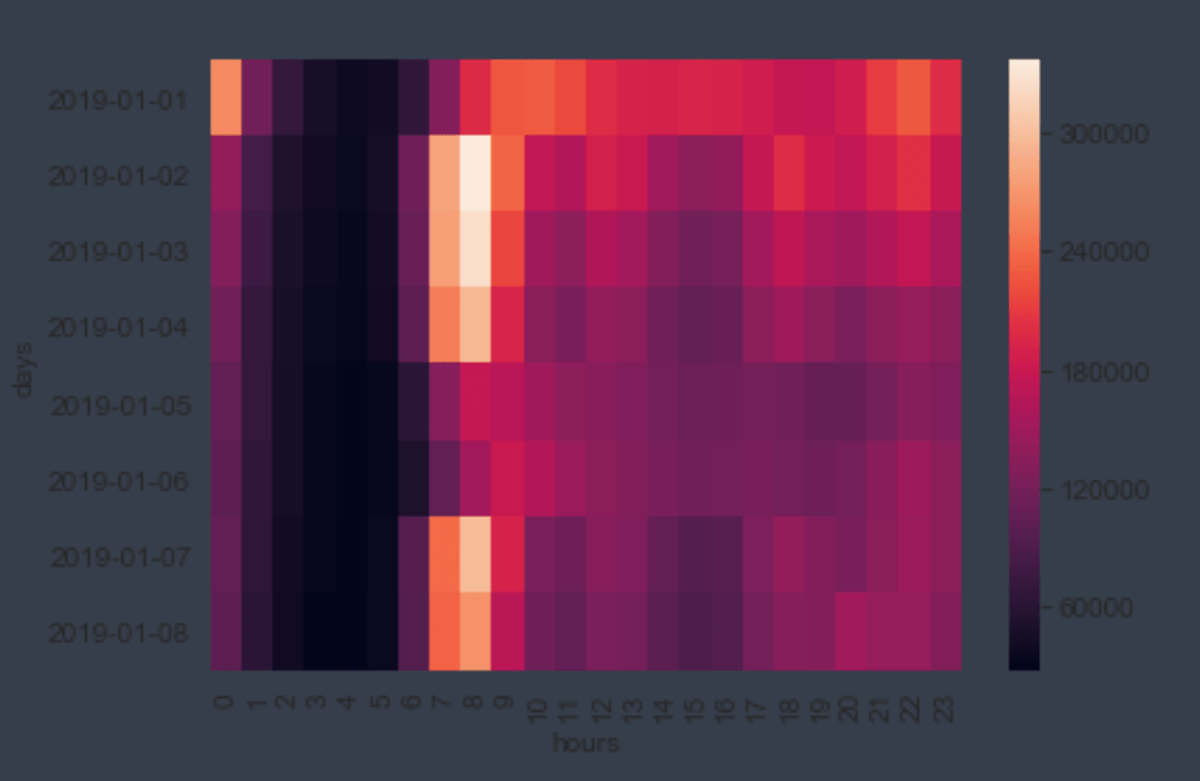# ggplot2 & seaborn

## 数据可视化技术

· 数据分析,数据可视化,R,Python

- R自不必说， 受本科翟老师（统计学院）影响，提前接触到了R语言。 ggplot2是本人现在还在使用 R Studio的唯一原因。

seaborn则是继 numpy\pandas\matplotlib 我的“Python数据分析三剑客”之后新学习的内容。 学习资料也来自于其home网址： https://seaborn.pydata.org

- 如果还没有安装的话， 分别执行

pip install numpy pandas matplotlib seaborn

==================================================================

install.packages(“ggplot2")

Simple Plot

（ seaborn提供了一些案例数据集， 在此基础上新增了几个jupyter notebook文件）>> sns.relplot(x="total_bill", y="tip", data=tip, hue="smoker") #same marker, differ colour

# differ marker differ colour

>> sns.relplot(x="total_bill", y="tip", data=tip, hue="smoker", style="smoker")

# differ range size ( 2,3,4...)

>> sns.relplot(x="total_bill", y="tip", data=tip, size="size", sizes=(15,200) )>> {r}

library(ggplot2)
# Set color to vary based on state categories.
gg <- ggplot(midwest, aes(x=area, y=poptotal)) +
geom_point(aes(col=state), size=3)

Complicated

Heatmap

```python

>>> flights = sns.load_dataset("flights") >>> flights = flights.pivot("month", "year", "passengers") >>> ax = sns.heatmap(flights)

```Reference

r-statistics 提供的ggplot2 tutorial

http://r-statistics.co/Complete-Ggplot2-Tutorial-Part1-With-R-Code.html

https://rud.is/b/2016/02/14/making-faceted-heatmaps-with-ggplot2/

ggplot2 参考

×